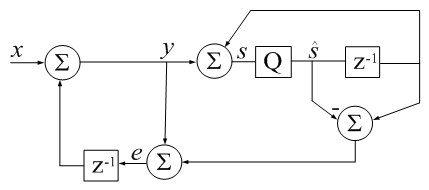# Accumulator Error Feedback

(Difference between revisions)
 Revision as of 19:15, 29 January 2018 (edit)← Previous diff Revision as of 19:28, 29 January 2018 (edit) (undo)Next diff → Line 16: Line 16: % Also see SUM. % Also see SUM. % % - % % Matlab csum() Example: + % Example: - % clear all + % csumv=0; rsumv=0; % csumv=0; rsumv=0; + % n = 100e6; + % t = ones(n,1); % while csumv <= rsumv % while csumv <= rsumv - % v = randn(13e6,1); + % v = randn(n,1); - % rsumv = abs(sum(v) - sum(v(end:-1:1))); + % - % disp(['rsumv = ' num2str(rsumv,'%18.16f')]); + % rsumv = abs((t'*v - t'*v(end:-1:1))/sum(v)); - % [~, idx] = sort(abs(v),'descend'); + % disp(['rsumv = ' num2str(rsumv,'%1.16f')]); - % x = v(idx); + % - % csumv = abs(csum(x) - csum(x(end:-1:1))); + % csumv = abs((csum(v) - csum(v(end:-1:1)))/sum(v)); - % disp(['csumv = ' num2str(csumv,'%18.16e')]); + % disp(['csumv = ' num2str(csumv,'%1.16e')]); % end % end

## Revision as of 19:28, 29 January 2018csum() in Digital Signal Processing terms: z-1 is a unit delay,
Q is a 64-bit floating-point quantizer. Algebra represents neither a sequence of instructions or algorithm. It is only meant to remind that an imperfect accumulator introduces noise into a series.
qi represents error due to quantization (additive by definition).
```function s_hat = csum(x)
% CSUM Sum of elements using a compensated summation algorithm.
%
% This Matlab code implements Kahan's compensated
% summation algorithm (1964) which often takes about twice as long,
% but produces more accurate sums when the number of
% elements is large. -David Gleich
%
% Also see SUM.
%
% Example:
% csumv=0;  rsumv=0;
% n = 100e6;
% t = ones(n,1);
% while csumv <= rsumv
%    v = randn(n,1);
%
%    rsumv = abs((t'*v - t'*v(end:-1:1))/sum(v));
%    disp(['rsumv = ' num2str(rsumv,'%1.16f')]);
%
%    csumv = abs((csum(v) - csum(v(end:-1:1)))/sum(v));
%    disp(['csumv = ' num2str(csumv,'%1.16e')]);
% end

s_hat=0; e=0;
for i=1:numel(x)
s_hat_old = s_hat;
y = x(i) + e;
s_hat = s_hat_old + y;
e = y - (s_hat - s_hat_old);
end
return
```

### sorting

Sorting is not integral above because the commented Example (inspired by Higham) would then display false positive results.
In practice, input sorting should begin the csum() function to achieve the most accurate summation:

```function s_hat = csum(x)
s_hat=0; e=0;
[~, idx] = sort(abs(x),'descend');
x = x(idx);
for i=1:numel(x)
s_hat_old = s_hat;
y = x(i) + e;
s_hat = s_hat_old + y;
e = y - (s_hat - s_hat_old);  %calculate parentheses first
end
return
```

Even in complete absence of sorting, csum() can be more accurate than conventional summation by orders of magnitude.